Theory of Computation | Generating regular expression from finite automata

There are two methods to convert FA to regular expression –
1. State Elimination Method –

• Step 1 –
If the start state is an accepting state or has transitions in, add a new non-accepting start state and add an €-transition between the new start state and the former start state.
• Step 2 –
If there is more than one accepting state or if the single accepting state has transitions out, add a new accepting state, make all other states non-accepting, and add an €-transition from each former accepting state to the new accepting state.
• Step 3 –
For each non-start non-accepting state in turn, eliminate the state and update transitions accordingly.

Example :-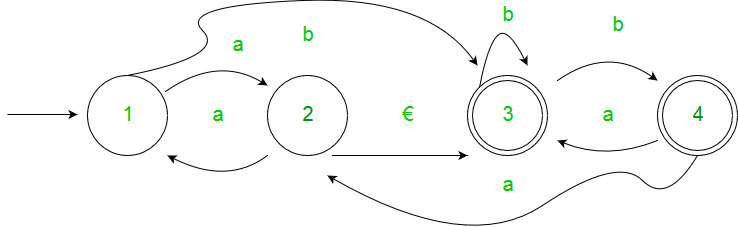Solution :-
Step 1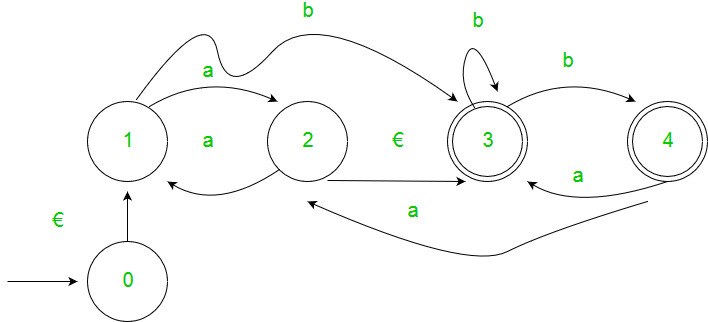Step 2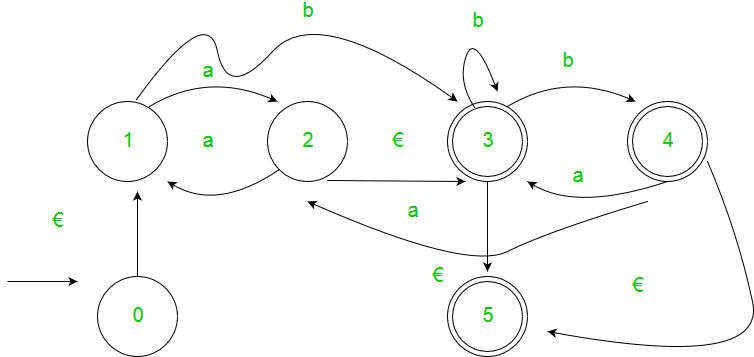Step 3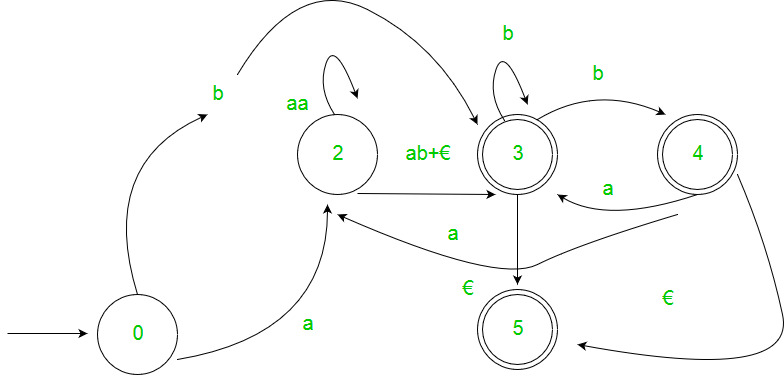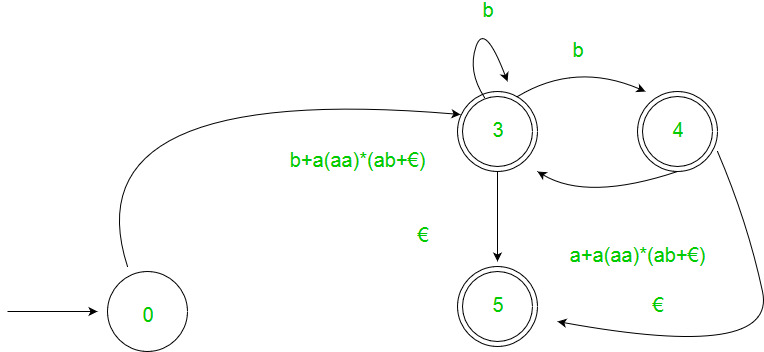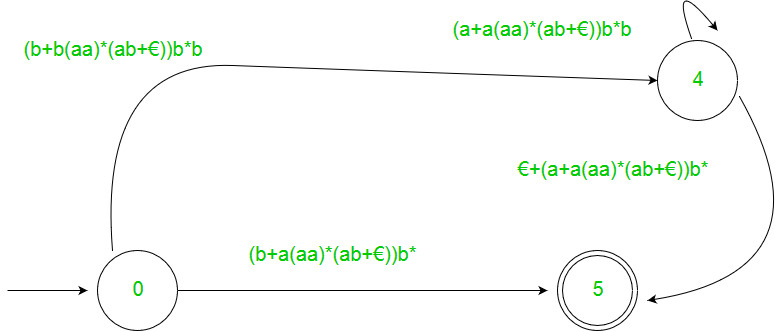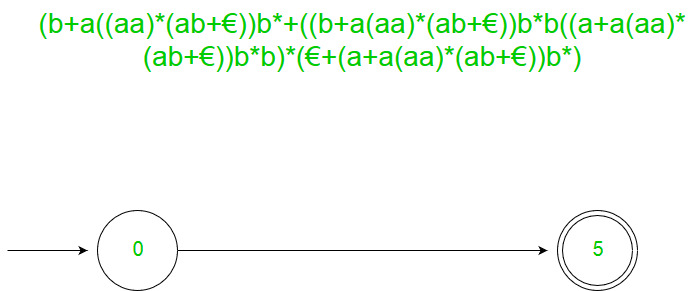2. Arden’s Theorem – Let P and Q be 2 regular expressions. If P does not contain null string, then following equation in R, viz R = Q + RP, Has a unique solution by R = QP*

Assumptions –

• The transition diagram should not have €-moves.
• It must have only one initial state.

Using Arden’s Theorem to find Regular Expression of Deterministic Finite automata –

1. For getting the regular expression for the automata we first create equations of the given form for all the states
q1 = q1w11 +q2w21 +…+qnwn1 +€ (q1 is the initial state)
q2 = q1w12 +q2w22 +…+qnwn2
.
.
.
qn = q1w1n +q2w2n +…+qnwnn

wij is the regular expression representing the set of labels of edges from qi to qj

Note – For parallel edges there will be that many expressions for that state in the expression.

2. Then we solve these equations to get the equation for qi in terms of wij and that expression is the required solution, where qi is a final state.

Example :-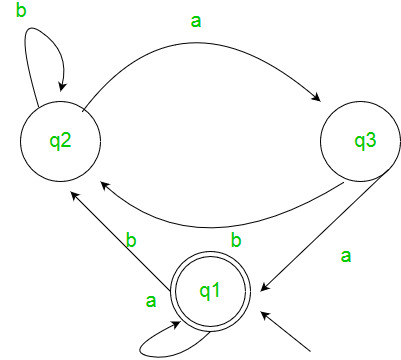Solution :-
Here the initial state is q2 and the final state is q1.
The equations for the three states q1, q2, and q3 are as follows ?
q1 = q1a + q3a + € ( € move is because q1 is the initial state)
q2 = q1b + q2b + q3b
q3 = q2a
Now, we will solve these three equations ?
q2 = q1b + q2b + q3b
= q1b + q2b + (q2a)b (Substituting value of q3)
= q1b + q2(b + ab)
= q1b (b + ab)* (Applying Arden’s Theorem)
q1 = q1a + q3a + €
= q1a + q2aa + € (Substituting value of q3)
= q1a + q1b(b + ab*)aa + € (Substituting value of q2)
= q1(a + b(b + ab)*aa) + €
= € (a+ b(b + ab)*aa)*
= (a + b(b + ab)*aa)*
Hence, the regular expression is (a + b(b + ab)*aa)*.

GATE CS Corner Questions

Practicing the following questions will help you test your knowledge. All questions have been asked in GATE in previous years or in GATE Mock Tests. It is highly recommended that you practice them.My Personal Notes arrow_drop_upCheck out this Author's contributed articles.

If you like GeeksforGeeks and would like to contribute, you can also write an article using contribute.geeksforgeeks.org or mail your article to contribute@geeksforgeeks.org. See your article appearing on the GeeksforGeeks main page and help other Geeks.

Please Improve this article if you find anything incorrect by clicking on the "Improve Article" button below.

Article Tags :

1

Please write to us at contribute@geeksforgeeks.org to report any issue with the above content.